﻿ Vibration and Parametric Instability of Functionally Graded Material Plates

### Vibration and Parametric Instability of Functionally Graded Material Plates

Ramu I, Mohanty SCOPEN ACCESSPEER-REVIEWED

## Vibration and Parametric Instability of Functionally Graded Material Plates

Ramu I1,, Mohanty SC1

1Department of Mechanical Engineering, NIT, Rourkela, India

### Abstract

The present work aims the vibration and parametric instability of functionally graded material rectangular plates with simply supported boundary condition, subjected to a biaxial in-plane periodic loading. First order shear deformation theory is used for theoretical formulation of FGM plates. The properties of the functionally graded material plates are assumed to vary along the thickness direction according to a power law distribution in terms of the volume fractions of the constituents. Hamilton’s principle is employed to convert the governing equations into a linear system of Mathieu–Hill equations from which the boundary of stable and unstable regions are determined by using Floquet’s theory on the parameter space. Natural frequency and buckling analysis are also discussed. Numerical results are presented in both dimensionless parameters and graphical forms for FGM plates made of steel and alumina. The influences of various parameters such as index value, aspect ratio on the buckling load and natural frequencies are examined. Power law index value and aspect ratio effects on the dynamic stability regions also studied in detail.

### At a glance: Figures

1234
Prev Next

• I, Ramu, and Mohanty SC. "Vibration and Parametric Instability of Functionally Graded Material Plates." Journal of Mechanical Design and Vibration 2.4 (2014): 102-110.
• I, R. , & SC, M. (2014). Vibration and Parametric Instability of Functionally Graded Material Plates. Journal of Mechanical Design and Vibration, 2(4), 102-110.
• I, Ramu, and Mohanty SC. "Vibration and Parametric Instability of Functionally Graded Material Plates." Journal of Mechanical Design and Vibration 2, no. 4 (2014): 102-110.

 Import into BibTeX Import into EndNote Import into RefMan Import into RefWorks

### 1. Introduction

Functionally graded materials (FGM) are designed in such way to overcome the demerits of ordinary materials. It is so called as FGM because the properties changes as thickness changes which is not observed in ordinary materials. These materials have many advantages such as high resistance to temperature gradients, high wear resistance, reduction in residual and thermal stresses, and an increase in strength to weight ratio because of these inherent properties stability is also increases. Due to the outstanding properties of Functionally graded materials (FGMs) are used in many engineering applications such as the aerospace, aircraft, automobile, defence, biomedical and electronic industries. Many structural components can be modelled as plates like structural. Plate like structures are often subjected to various types of dynamic loads, among which the periodically in-plane time-varying force may cause dynamic instability, in which there is an unbounded exponential built up of the response. In practice the dynamic loads are dependent on time and may change their direction. It is enormous practical importance to clarify the dynamic stability of dynamic systems under periodic loads. Therefore, a broad understanding of the dynamic stability characteristics of structural materials in periodic loading environments is a matter of important weight for the design of the structural failure.

FGMs are made of a ceramic and a metal in such a way that the ceramic can resist the thermal loading from the high temperature environment. The material properties of FGMs vary continuously from one surface to the other surface, this results in eliminating surface problems of composite materials and achieving the smooth stress distribution. Theoretical modelling and analysis of FGM plates has become important topic to discussion at the present stage. The classical laminated plate theory has become the most widespread engineering application, a lot of articles about FGM plates and shells based on the classical laminated plate theory were reported. The recent advancement in the characterization, modelling and analysis of FGM has been reviewed Victor Birman and Larry  in this work focussed on research published since 2000. Due to the broad and rapidly developing field to various aspects of theory and applications of FGM are reflected in this effort. They reflect some of the observations of the authors based on the published research and their own analysis of the subject on FGM. The physical neutral surface and geometric middle surface are the same in homogenous symmetrical plates. The concept of neutral surface was derived based on the classical nonlinear plate theory for the FGM plates by Da-Guang and You-He . Theoretical formulation and finite element modals for functionally graded plates based on the third-order shear deformation theory presented by Reddy . In this the finite element model that accounts for the thermo mechanical coupling and geometric non-linearity.

Free vibration analysis of FGM rectangular plates has been numerically performed by number of researchers. Zhao X. et al.  have studied a method for analyzing the free vibration of FGPs with arbitrary boundary conditions using the element-free kp-Ritz method. In their analysis a mesh-free kernel particle functions ware used to approximate the two-dimensional displacement fields. Refined two-dimensional shear deformation theory investigated by Fares et al.  for orthotropic FG Plates. For obtaining this theory a modified version of the mixed variational principle of Reissner was used. This theory does not require any shear correction factor. An exact analytical solution was developed by Hasani Baferani et al.  for free vibration analysis of thin FG rectangular plates by using the classical plate theory. In their analysis the effects of in-plane displacement on the vibration of FG rectangular plates are studied and also a closed-form solution for finding the natural frequency of FG simply supported rectangular plates. A 2-D higher-order theory is developed by Hiroyuki  for analyzing natural frequencies and buckling stresses of FG plates. Here the Hamilton’s principle was used for dynamic analysis of a two-dimensional (2-D) higher-order theory for rectangular functionally graded (FG) plates. A finite element method (FEM) of B-spline wavelet on the interval (BSWI) was used to solve the free vibration and buckling analysis of plates by Zhibo Yang et al. . In their analysis BSWI functions are considered for Structural analysis, the proposed method can obtained a fast convergence and a satisfying numerical accuracy with fewer degrees of freedoms (DOF). Serge  performing a direct analysis for natural frequencies of FG plates can be easily calculated from those known isotropic material results. Senthil and Batra  investigated an exact solution for the vibration of simply supported rectangular thick plates. They assumed that the plate is made of an isotropic material with material properties varying in the thickness direction only.

The paper conducts the vibration and parametric instability of functionally grade material plates under in-plane time-varying pulsating force. Four node rectangular elements are used for modelled as the FGM plate by using finite element method. Hamilton’s principle is employed to convert the governing equations into a linear system of Mathieu–Hill equations from which the boundary of stable and unstable regions are determined by using Floquet’s theory. For this analysis steel and alumina materials are used to make the FGM plate. Free vibration and static stability analysis are also discussed as parting problems. Numerical analyses are presented in both dimensionless parameters and graphical forms. The influences of various parameters on parametric instability of FGM plate studied in detail.

### 2. Methodology

2.1. Formulation of the problem

The FGM plate is of uniform rectangular cross-section having a length L, width W and thickness h. The plate is subjected to a pulsating axial force, acting along its axial axis. Ω is the excitation frequency of the dynamic load component, Ps is the static and Pt is the amplitude of the time dependent component of the load. A typical FGM plate subjected to in plane biaxial in plane dynamic loads as show in Figure 1.

Figure 1. FGM plate subjected to in plane biaxial in plane dynamic loads

The extensive use of plates the various types of functionally graded material plates was considerable interests to many researchers in the field of modelling, analysis and design of these structures. Accurate prediction of structural response characteristics is a demanding problem for the analysis of functionally graded materials due to the anisotropic structural behaviour and the presence of various types of complicated constituents. This is possible because the material composition of an FGM changes gradually through-the-thickness. The graphical representation Vc and Vm (volume fraction of ceramic and metal) of FGM through along the thickness direction of the specimen.

2.3. The Simple Power Law

More common in the analysis for functionally graded materials with two constituent materials the variations through the thickness of material properties P can be expressed as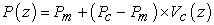(1)

Here P can represent Young's modulus E, Poisson ratio μ, and the mass density ρ, and Vc (z) is the volume fraction variation of the ceramic material, and it is assumed to follow a simple power-law distribution as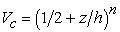(2)

Where -h/ 2≤ z ≤ h/ 2 is coordinate through the thickness from the middle surface to ceramic and metal sides, and n is a gradient index. Working range of design requirements in this case is based on a grading indexed.

2.4. Physical Neutral Surface of the FGM Plate

In the present work neutral axis concept has been employed for analysis. For a FGM plate due to the variation of the material properties along thickness, the neutral plane does not coincide with the geometrical mid-plane of the plane shown in Figure 2. The distance of the neutral surface (d) from the geometric mid-surface may be expressed as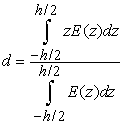(3)
2.5. Kinematics

Plate structures made of functionally graded materials are characterized by first order shear deformation theory so that the extension of the classical model suggested by (Reissner and Mindlin 2004) to the case of graded material plates provides a good compromise between numerical accuracy and computational load. In-plane displacements u and v and the normal displacement w are therefore given the form: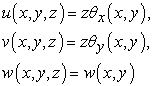(4)

where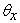and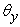are the rotations of the normal to the undeformed middle surface in the x–z and y–z planes, respectively.

In-plane and out-of-plane strain-displacement constitutive law relations may written as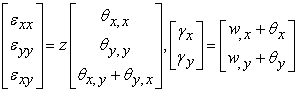(5)

The normal stress-strain and shear stress-strain relationships of the functionally graded plate in the global x-y coordinates system can be written as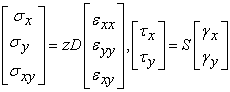(6)

whereas grading matrices D and S are defined as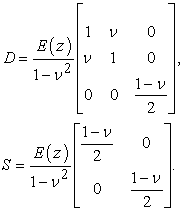Moments and shear forces are obtained via standard integration over the thickness, i.e.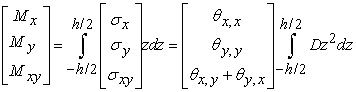(7a)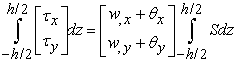(7b)
2.6. Energy equations

The potential strain energy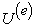of the plate can be written in terms of the stress resultants and strain components through integrating by parts with respect to coordinates as follows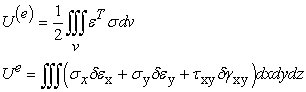(8)

The kinetic energy of the plate follows as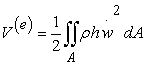(9)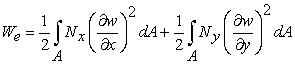(10)

where Nx and Ny are the in-plane compression loads.

2.7. FE formulation of a 4-noded Rectangular Element

Rectangular four node element is having one node at each corner as shown in Figure 5. There are three degrees of freedom at each node, the displacement component along the thickness (w), and two rotations along X and Y directions in terms of the (x, y) coordinates (Ramu I and Mohanty S C 2012). The element consists of four nodes 1, 2, 3 and 4 with w, θx and θy as the w is the transverse displacement and θx and θy represents the rotations about x and y axis.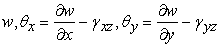(11)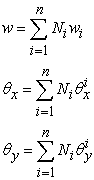(12)

Here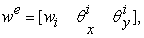Ni displacement vector and the appropriate shape functions, n number of nodes in the element.

The element stiffness matrix, geometric stiffness and mass matrices are derived on the basis of principle of minimum potential, kinetic energy and work done.

The element stiffness matrix is derived as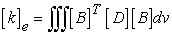(13)

where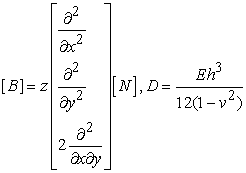Element mass matrix is derived as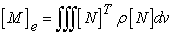(14)

The element geometric stiffness matrix is derived as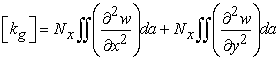(15)

### 3. Governing Equations of Motion

The element equation of motion for a plate subjected to axial force is obtained by using Hamilton’s principle.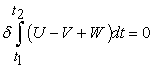(16)

By dividing the plate in to number of elements and assembling the element matrices, the potential energy and the kinetic energy for element can be written in terms of global displacement vector as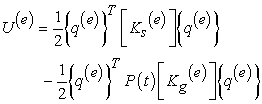(17)

Here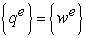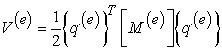(18)

The equation of motion of plate element in matrix form for the axially loaded discretised system is obtained as follows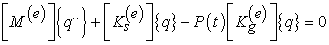(19)

The governing equation of motion of plate in terms of global displacement matrix obtained as follows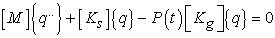(20)

Here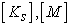and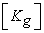are global stiffness, global mass and geometric stiffness matrix respectively.

Where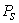is the static and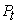the amplitude of time dependent component of the load, can be represented as function of fundamental static buckling load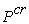of the plate and having all sides simply supported boundary conditions. Hence substituting,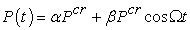with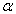and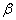are called static and dynamic load factors respectively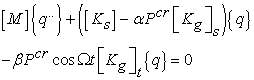(21)

Where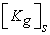and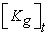reflect the influence of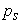and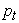respectively. If the static and time dependent components of the load are applied in the same manner then,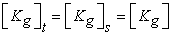3.1. Parametric Instability Regions

From the above equation (21) represents a system of second order differential equations with periodic coefficients the Mathieu-Hill type. From the theory of Mathieu function is evident that the nature of solution is dependent on the choice of load frequency and load amplitude. The frequency amplitude domain is divided in two regions, which give raise to stable solutions and to regions, which cause unstable solutions. According to the Floquet’s theory the periodic solutions characterize the boundary conditions between the dynamic stability and instability zones. So the periodic solution can be expressed as Fourier series.

A solution with period 2T is represented by:

The boundaries of the principal instability regions with period 2T are of practical importance.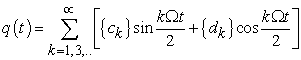(22)

If the series expansions of eq. (22), term wise comparisons of the sine and cosine coefficients will give infinite system of homogeneous algebraic equations for the vectors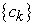and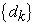for the solutions on the stability borders. Non-trivial solutions exist if the determinant of the coefficient matrices of these equation systems of infinite order vanishes.

Substituting the first order (k=1) Fourier series expansion of eq. (22) in eq. (21) and comparing the coefficients of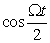and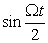terms, the condition for existence of these boundary solutions with period 2T is given by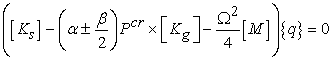(23)

The above equation represents an eigenvalue problem for known values ofand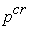. This equation gives two sets of eigenvalues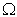bounding the regions of stability due to the presence of plus and minus sign. The instability boundaries can be determined from the solution of the equation.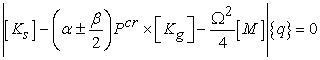(24)

Also the equation represents the solution to a number of related problems

(1) For natural frequencies: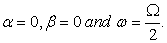The equation becomes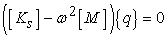(25)

(2) For static stability or buckling analysis: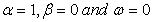The equation becomes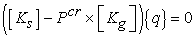(26)

(3) For dynamic instability, when all terms are present

Let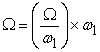Where the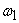is the fundamental frequency of the plate having the different boundary conditions, equation then becomes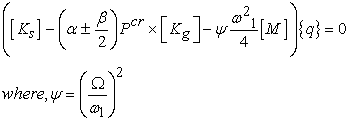(27)

The region of parametric instability can be determined by using equation (27).

### 4. Results and Discussions

4.1. Validation of Results

The results for FGM plate free vibration and buckling analysis obtained by applying first order shear deformation theory in this study are compared by the ref. results Talha & Singh . The natural frequencies are obtained by considering a combination of Al/ZrO2 and SUS304/Si3N4 where the top surface is ceramic rich and the bottom surface is metal rich. In according to the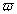the dimensionless frequency parameter from Talha & Singh  is: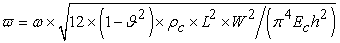where Ec and ρc are young’s modulus and density of ceramic material. Validation has been done by considering the values of thickness, length, width, Poisson’s ratio, density and young’s modulus in the ceramic and metal as:

Al, ρ=2702kg/m3, E=70109Pa, ϑ =0.3

ZrO2, ρ=3,000kg/m3, E=151109Pa, ϑ =0.3

SUS304, ρ=8,166kg/m3, E=207.78109Pa, ϑ=0.3177

Si3N4, ρ=2,370kg/m3, E=322.27109Pa, ϑ=0.24

Table 1 and 2 shows the natural frequency parameter obtained from the present study using first order shear deformation theory and Talha & Singh . There is a good matching between the presented results and those from ref. , particularly for simply supported case. There is less difference between the results predicted by first order shear deformation theory and higher order theories.

#### Table 3. Comparison of the critical buckling load (MN/m) for a FGM plate (L = 1, h = 0.01)

FGM plate buckling analysis has been compared and studied. The buckling analysis is performed for FGM rectangular plate’s different values of power law index, for aluminium-alumina FGM. The Young’s modulus and Poisson’s ratio for aluminium are: 70 GPa and 0.3 and for alumina: 380 GPa and 0.3, respectively. To validate the derived equation, the obtained critical buckling loads of simply supported FGM plates shown in Table 3. They are good matching between the calculated and results of Mokhtar et al.  for low values of L/h.

4.2. Numerical Results
4.2.1. Natural Frequency and Buckling Analysis

The following numerical results are obtained by considering the steel as the bottom surface and alumina as the top surface in FGM plate according to index value. The geometry of plate and material properties is as follows:

L=1 m (length), W=1 m (width), h=0.1 m (thickness).

SUS304, ρ=7,800kg/m3, E=201109Pa, ϑ=0.3

Al2O3, ρ=2,707kg/m3, E=380109Pa, ϑ=0.3.

The variations of natural frequency parameter in FGM (SUS304/Al2O3) plate with different boundary conditions are shows in Figure 6 and Figure 7. The effect of power law index n on the frequencies can be seen by different boundary conditions. As expected, the increasing index value leads to reduce the natural frequency. The increase power law index reduces the ceramic volume fraction; it affects the effective material properties.

In Figure 8 shows the results of critical buckling load of a FGM rectangular plate based CPT was presented. This figure shows that the critical buckling load decreases when the power law index value increases.

4.2.2. Dynamic Stability Analysis

The dynamic stability of FGM plates under parametric excitation was investigated. The power law index value, the length, the width and the thickness of the FGM plates were varied to assess their effects on the parametric instability behaviour of the FGM plates. Figure 9 shows the dynamic stability of simply supported FGM plate aspect ratio L/W=0.5, 1, 1.5 and plate thickness h=0.1m. The effects of aspect ratios on the first three instability regions for simply supported plate are presented in Figure 9a, Figure 9b, Figure 9c. Increase the aspect ratio L/W the unstable region is located father from dynamic load axis, it shows that reduces the dynamic instability of simply supported rectangular FGM plate. Figure 10a, Figure 10b and Figure 10c shows the first three parametric instability regions of FGM plate of rectangular cross-section with various index values and aspect ratio is examined. It can be seen that the instability regions are shifted towards the dynamic load axis with increases power law index value at lower excitation frequency. The effect is much more significant on another three instability regions than on the first region. Increase in power law index increases the dynamic stability.

Figure 10. Dynamic stability regions for simply supported FGM plate with different index values n=1, 2, 3

### 5. Conclusions

Finite element modelling of rectangular FGM plate has been developed using first order shear deformation theory. Based on the above formulation various types of analyses i.e. free vibration, buckling and dynamic stability have been carried out. In case of FGM plate with increase of power law index value, the first five natural frequencies decrease. If L/W ratio is increased the critical buckling load decreases for each case of loading and it is also observed that as the power law index value increases critical buckling load decreases. FGM plate vibration and static characteristics can be improved by developing proper material grading. Increase in aspect ratio L/W=0.5, 1 and 1.5 of rectangular plate results in overall enhancement of instability of the plate. With increase of the power index value n=1, 2 and 3 instability regions moves closer to dynamic load axis with the different aspect ratios, it shows that there is deterioration of the dynamic stability.

### References

  Victor, B. and Larry, W. B, “Modelling and Analysis of Functionally Graded Materials and Structures,” ASME Applied Mechanics Reviews, 60. 195-203. Sep. 2007.In article CrossRef  Da-Guang, Z. and You-He, Z, “A theoretical analysis of FGM thin plates based on physical neutral surface,” Computational Materials Science, 44. 716-720. 2008.In article CrossRef  Reddy, J.N, “Analysis of functionally graded plates,” International Journal of Numerical Methods in Engineering, 47. 663-684. 2000.In article CrossRef  Zhao, X., Lee, Y.Y. and Liew, K.M, “Free vibration analysis of functionally graded plates using the element-free kp-Ritz method,” Journal of Sound and Vibration, 319. 918-939. 2009.In article CrossRef  Fares, M.E, Elmarghany M.K. and Doaa, A, “An efficient and simple refined theory for bending and vibration of functionally graded plates,” Composite Structures, 91. 296-305. 2009.In article CrossRef  Hasani, B., Saidi, A.R. and Jomehzadeh, E, “An exact solution for free vibration of thin functionally graded rectangular plates,” Proceedings IMechE Part C: Journal of Mechanical Engineering Science, 225. 526-536. 2010.In article CrossRef  Hiroyuki, M, “Free vibration and stability of functionally graded plates according to a 2-D higher-order deformation theory,” Composite Structures, 82. 499-512. 2008.In article CrossRef  Zhibo, Y., Xuefeng, C., Xingwu, Z., Zhengjia, H, “Free vibration and buckling analysis of plates using B-spline wavelet on the interval Mindlin element,” Applied Mathematical Modelling, 07. 55-64. 2012.In article  Serge, A, “Free vibration, buckling and static deflections of functionally graded plates,” Composites Science and Technology, 66. 2383-2394. 2006.In article CrossRef  Senthil, S.V. and Batra, R.C, “Three-dimensional exact solution for the vibration of functionally graded rectangular plates,” Journal of Sound and Vibration, 272. 703-730. 2004.In article CrossRef  Hosseini-Hashemi, Sh., Fadaee, M., Atashipour, S.R, “Study on the free vibration of thick functionally graded rectangular plates according to a new exact closed-form procedure,” Composite Structures, 93. 722-735. 2011.In article CrossRef  Mohammad, T. and Singh, B.N, “Static response and free vibration analysis of FGM plates using higher order shear deformation theory,” Applied Mathematical Modelling, 34. 3991-4011. 2010.In article CrossRef  Ferreira, A.J.M., Batra, R.C., Roque, C.M.C., Qian, L.F. and Jorge, R.M.N, “Natural frequencies of functionally graded plates by a meshless method,” Composite Structures, 75. 593-600. 2006.In article CrossRef  Suresh Kumar, J., Sidda Reddy, Eswara Reddy, C. and Vijaya Kumar Reddy, K, “Higher Order Theory for Free Vibration Analysis of Functionally Graded Material Plates,” ARPN Journal of Engineering and Applied Sciences, 10(6). 105-111. 2011.In article  Mohammad, T. and Singh, B.N, “Static response and free vibration analysis of FGM plates using higher order shear deformation theory,” Applied mathematical modelling, 34. 3991-4011. 2010.In article CrossRef  Rohit, S. and Maiti, P.R, “Buckling of simply supported FGM plates under uniaxial load,” International Journal of Civil and Structural Engineering, 2(4). 1035-1050. 2012.In article  Xinwei, W., Lifei, G., Yihui, Z, “Differential quadrature analysis of the buckling of thin rectangular plates with cosine-distributed compressive loads on two opposite sides,” Advances in Engineering Software, 39. 497-504. 2008.In article CrossRef  Mokhtar, B., Djamel, O., Abdelaziz, Y. and Abdelmadjid, H, “Buckling of Thin Plates under Uniaxial and Biaxial Compression,” Journal of Materials Science and Engineering B, 2 (8). 487-492. 2012.In article  Andrzej, T, “Dynamic Stability of Functionally Graded Plate under In-Plane Compression,” Mathematical Problems in Engineering, 4. 411-424. 2005.In article  Ramu, I. and Mohanty, S.C, “A Review on Free, Forced Vibration Analysis and Dynamic Stability of Ordinary and Functionally Grade Material Plates,” Caspian Journal of Applied Sciences Research, 1(13). 57-70. 2012.In article  Ramu, I. and Mohanty, S.C, “Study on Free Vibration Analysis of Rectangular Plate Structures Using Finite Element Method,” 2nd ICMOC-Hyderabad, Elsevier Procardia Engineering, 38. 2758-2766. 2012.In article  Lucia, D.C., Paolo, V, “Finite elements for functionally graded Reissner–Mindlin plates,” Computer Methods in Applied Mechanics and Engineering, 193. 705-725. 2004.In article CrossRef  Ng, T.Y., Lam, K.Y., Liew, K.M., Reddy, J.N, “Dynamic stability analysis of functionally graded cylindrical shells under periodic axial loading,” International Journal of Solids and Structures, 38. 1295-1309. 2001.In article CrossRef  Bolotin, V.V, The dynamic stability of elastic systems volume I, report, Aerospace Corporation, EI Segundo, California. 1962.In article  Rath, M.K. and Dash. M.K., Parametric Instability of Woven Fiber Laminated Composite Plates in Adverse Hygrothermal Environment. American Journal of Mechanical Engineering, 2(3). 70-81. 2014.In article CrossRef